## Selina Concise Mathematics Class 10 ICSE Solutions Chapter 10 Arithmetic Progression Ex 10D

These Solutions are part of Selina Concise Mathematics Class 10 ICSE Solutions. Here we have given Selina Concise Mathematics Class 10 ICSE Solutions Chapter 10 Arithmetic Progression Ex 10D

Other Exercises

Question 1.
Find three numbers in A.P. whose sum is 24 and whose product is 440.
Solution:
Let three numbers be a – d, a, a + d
a – d + a + a + d = 24Question 2.
The sum of three consecutive terms of an A.P. is 21 and the slim of their squares is 165. Find these terms.
Solution:
Let three consecutive numbers in A.P. are
a – d, a, a + d
a – d + a + a + d = 21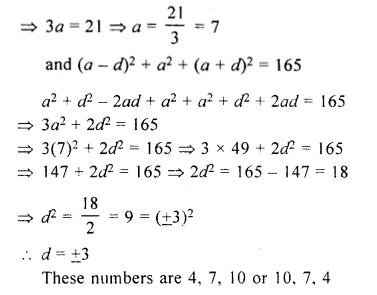Question 3.
The angles of a quadrilateral are in A.P. with common difference 20°. Find its angles.
Solution:
Let the angles of a quadrilateral are
a, a + d, a + 2d, a + 3d
d= 20°
a + a + d + a + 2d + a + 3d = 360°
(Sum of angles of a quadrilateral)
=> 4a + 6d = 360°
=> 4a + 6 x 20° = 360°
=> 4a + 120° = 360°
=> 4a = 360 – 120° = 240°
a = $$\\ \frac { 240 }{ 4 }$$ = 60°
Angles are 60°, 80°, 100°, 120°

Question 4.
Divide 96 into four parts which are in A.P. and the ratio between product of their means to product of their extremes is 15 : 7.
Solution:
Number = 96
Let its four parts be a, a + d, a + 2d, a + 3d
a + a + d + a + 2d + a + 3d = 96
=> 4a + 6d = 96
=> 2a + 3d = 48 …(i)
Product of means : Product of extremes = 15 : 7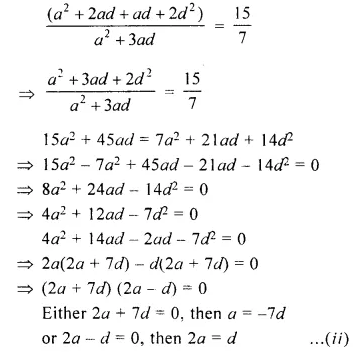Question 5.
Find five numbers in A.P. whose sum is $$12 \frac { 1 }{ 2 }$$ and the ratio of the first to the last terms is 2 : 3.
Solution:
Let 5 numbers in A.P. be
a, a + d, a + 2d, a + 2d, a + 4d
a + a + d + a + 2d + a + 3d + a + 4d =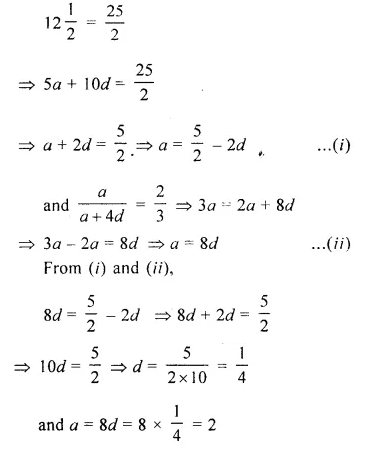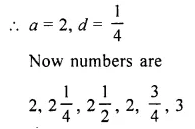Question 6.
Split 207 into three parts such that these parts are in A.P. and the product of the two smaller parts is 4623.
Solution:
Number = 207
Let part be a – d, a, a + d
a – d + a + a + d = 207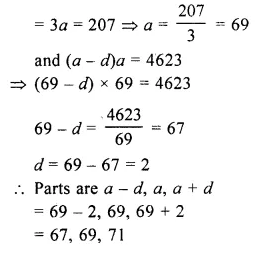Question 7.
The sum of three numbers in A.P. is 15 the sum of the squares of the extreme is 58. Find the numbers
Solution:
Let three numbers in A.P. be a – d, a, a + d
a – d + a + a + d = 15
=> 3a = 15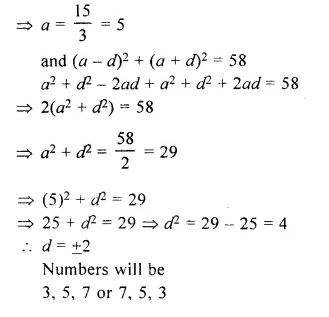Question 8.
Find four numbers in A.P. whose sum is 20 and the sum of whose squares is 120.
Solution:
Let four numbers in A.P. be
a – 3d, a – d, a + d, a + 3d
a – 3d + a – d + a + d + a + 3d = 20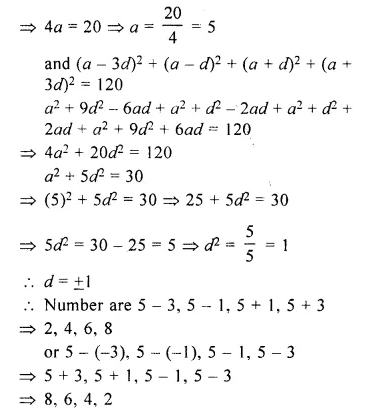Question 9.
Insert one arithmetic mean between 3 and 13.
Solution:
Let A be the arithmetic mean between 3 and 13
$$\left( A=\frac { a+b }{ 2 } \right)$$
A = $$\\ \frac { 3+13 }{ 2 }$$
= $$\\ \frac { 16 }{ 2 }$$
= 8

Question 10.
The angles of a polygon are in A.P. with common difference 5°. If the smallest angle is 120°, find the number of sides of the polygon.
Solution:
Angles of a polygon are in A.P.
and common difference (d) = 5°
Smallest angle (a) = 120°
Let n be the number of sides of the polygon then sum of angles = (2n – 4) x 90°
a = 120° and d = 5°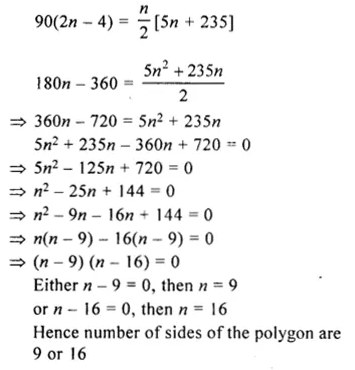Question 11.
$$\\ \frac { 1 }{ a }$$, $$\\ \frac { 1 }{ b }$$ and $$\\ \frac { 1 }{ c }$$ are in A.P
S.T : bc, ca and ab are also in A.P
Solution:
$$\\ \frac { 1 }{ a }$$, $$\\ \frac { 1 }{ b }$$ and $$\\ \frac { 1 }{ c }$$ are in A.P
We have to show thatHope given Selina Concise Mathematics Class 10 ICSE Solutions Chapter 10 Arithmetic Progression Ex 10D are helpful to complete your math homework.

If you have any doubts, please comment below. Learn Insta try to provide online math tutoring for you.